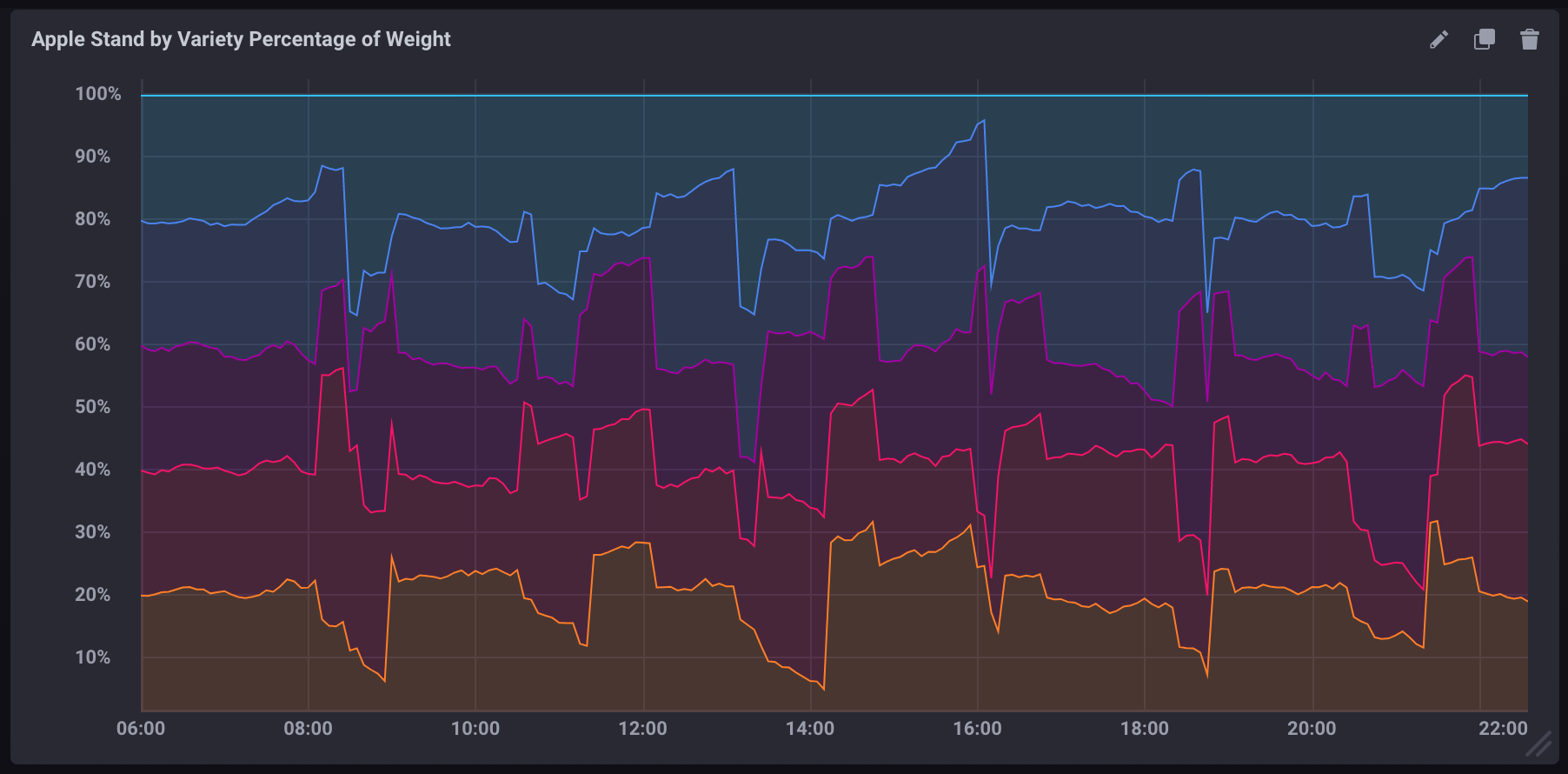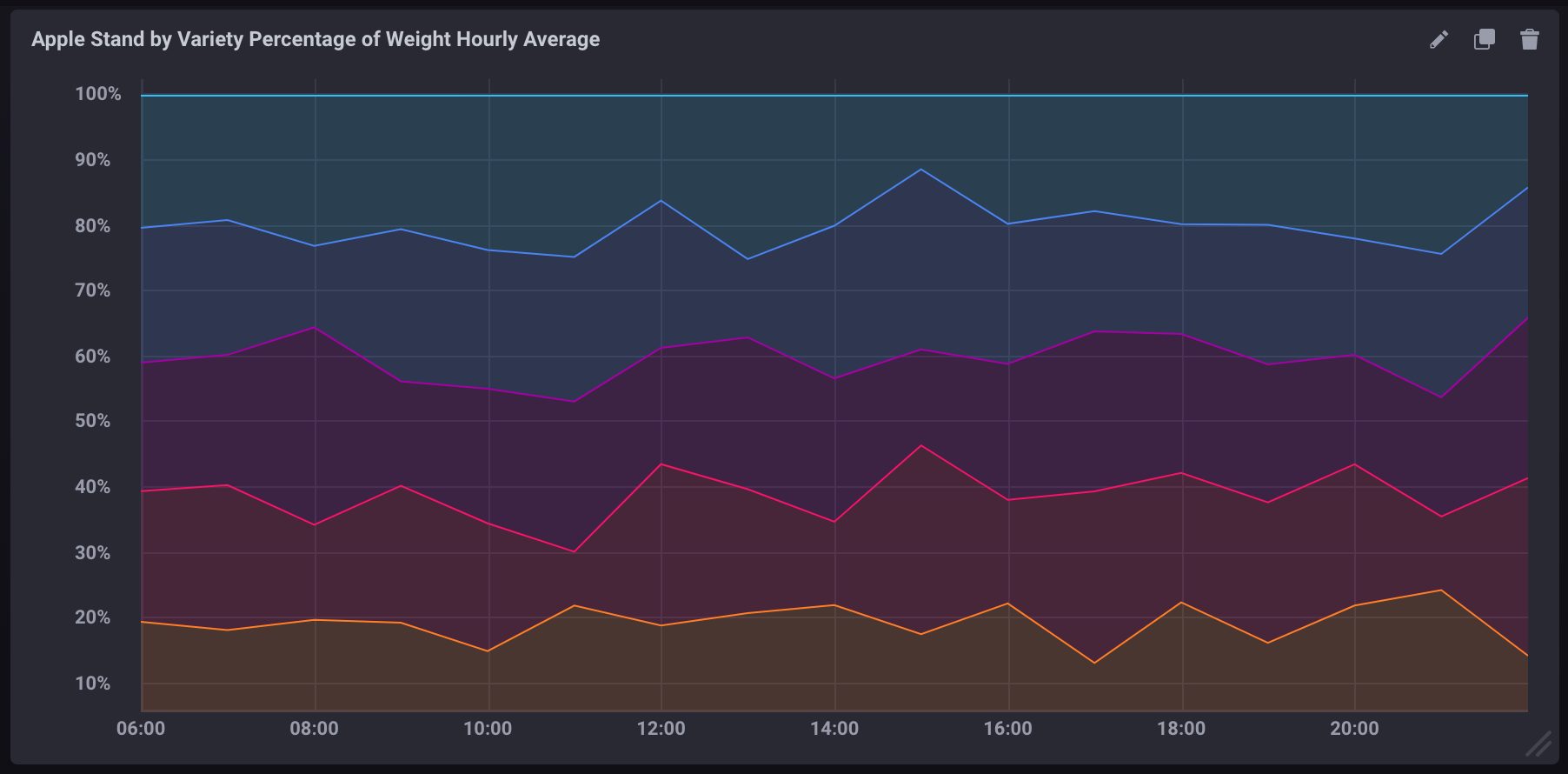# Calculate percentages in a query

This page documents an earlier version of InfluxDB. InfluxDB v2.6 is the latest stable version. See the equivalent InfluxDB v2.6 documentation: Calculate percentages with Flux.

Use Flux or InfluxQL to calculate percentages in a query.

Flux lets you perform simple math equations, for example, calculating a percentage.

## Calculate a percentage

Learn how to calculate a percentage using the following examples:

## Basic calculations within a query

When performing any math operation in a Flux query, you must complete the following steps:

1. Specify the bucket to query from and the time range to query.
2. Filter your data by measurements, fields, and other applicable criteria.
3. Align values in one row (required to perform math in Flux) by using one of the following functions:

For examples using the `join()` function to calculate percentages and more examples of calculating percentages, see Calculate percentages with Flux.

#### Data variable

To shorten examples, we’ll store a basic Flux query in a `data` variable for reuse.

Here’s how that looks in Flux:

``````// Query data from the past 15 minutes pivot fields into columns so each row
// contains values for each field
data = from(bucket:"your_db/your_retention_policy")
|> range(start: -15m)
|> filter(fn: (r) =>  r._measurement == "measurement_name" and r._field =~ /field[1-2]/)
|> pivot(rowKey:["_time"], columnKey: ["_field"], valueColumn: "_value")
``````

Each row now contains the values necessary to perform a math operation. For example, to add two field keys, start with the `data` variable created above, and then use `map()` to re-map values in each row.

``````data
|> map(fn: (r) => ({ r with _value: r.field1 + r.field2}))
``````

Note: Flux supports basic math operators such as `+`,`-`,`/`, `*`, and `()`. For example, to subtract `field2` from `field1`, change `+` to `-`.

## Calculate a percentage from two fields

Use the `data` variable created above, and then use the `map()` function to divide one field by another, multiply by 100, and add a new `percent` field to store the percentage values in.

``````data
|> map(fn: (r) => ({
_time: r._time,
_measurement: r._measurement,
_field: "percent",
_value: field1 / field2 * 100.0
}))
``````

Note: In this example, `field1` and `field2` are float values, hence multiplied by 100.0. For integer values, multiply by 100 or use the `float()` function to cast integers to floats.

## Calculate a percentage using aggregate functions

Use `aggregateWindow()` to window data by time and perform an aggregate function on each window.

``````from(bucket:"<database>/<retention_policy>")
|> range(start: -15m)
|> filter(fn: (r) =>  r._measurement == "measurement_name" and r._field =~ /fieldkey[1-2]/)
|> aggregateWindow(every: 1m, fn:sum)
|> pivot(rowKey:["_time"], columnKey: ["_field"], valueColumn: "_value")
|> map(fn: (r) => ({ r with _value: r.field1 / r.field2 * 100.0 }))
``````

## Calculate the percentage of total weight per apple variety

Use simulated apple stand data to track the weight of apples (by type) throughout a day.

2. Import the sample data:
``````influx -import -path=path/to/apple_stand.txt -precision=ns -database=apple_stand
``````

Use the following query to calculate the percentage of the total weight each variety accounts for at each given point in time.

``````from(bucket:"apple_stand/autogen")
|> range(start: 2018-06-18T12:00:00Z, stop: 2018-06-19T04:35:00Z)
|> filter(fn: (r) =>  r._measurement == "variety")
|> pivot(rowKey:["_time"], columnKey: ["_field"], valueColumn: "_value")
|> map(fn: (r) => ({ r with
granny_smith: r.granny_smith / r.total_weight * 100.0 ,
golden_delicious: r.golden_delicious / r.total_weight * 100.0 ,
fuji: r.fuji / r.total_weight * 100.0 ,
gala: r.gala / r.total_weight * 100.0 ,
braeburn: r.braeburn / r.total_weight * 100.0 ,}))
``````

## Calculate the average percentage of total weight per variety each hour

With the apple stand data from the prior example, use the following query to calculate the average percentage of the total weight each variety accounts for per hour.

``````from(bucket:"apple_stand/autogen")
|> range(start: 2018-06-18T00:00:00.00Z, stop: 2018-06-19T16:35:00.00Z)
|> filter(fn: (r) => r._measurement == "variety")
|> aggregateWindow(every:1h, fn: mean)
|> pivot(rowKey:["_time"], columnKey: ["_field"], valueColumn: "_value")
|> map(fn: (r) => ({ r with
granny_smith: r.granny_smith / r.total_weight * 100.0,
golden_delicious: r.golden_delicious / r.total_weight * 100.0,
fuji: r.fuji / r.total_weight * 100.0,
gala: r.gala / r.total_weight * 100.0,
braeburn: r.braeburn / r.total_weight * 100.0
}))
``````

InfluxQL lets you perform simple math equations which makes calculating percentages using two fields in a measurement pretty simple. However there are some caveats of which you need to be aware.

## Basic calculations within a query

`SELECT` statements support the use of basic math operators such as `+`,`-`,`/`, `*`, `()`, etc.

``````-- Add two field keys
SELECT field_key1 + field_key2 AS "field_key_sum" FROM "measurement_name" WHERE time < now() - 15m

-- Subtract one field from another
SELECT field_key1 - field_key2 AS "field_key_difference" FROM "measurement_name" WHERE time < now() - 15m

-- Grouping and chaining mathematical calculations
SELECT (field_key1 + field_key2) - (field_key3 + field_key4) AS "some_calculation" FROM "measurement_name" WHERE time < now() - 15m
``````

## Calculating a percentage in a query

Using basic math functions, you can calculate a percentage by dividing one field value by another and multiplying the result by 100:

``````SELECT (field_key1 / field_key2) * 100 AS "calculated_percentage" FROM "measurement_name" WHERE time < now() - 15m
``````

## Calculating a percentage using aggregate functions

If using aggregate functions in your percentage calculation, all data must be referenced using aggregate functions. You can’t mix aggregate and non-aggregate data.

All Aggregate functions need a `GROUP BY time()` clause defining the time intervals in which data points are grouped and aggregated.

``````SELECT (sum(field_key1) / sum(field_key2)) * 100 AS "calculated_percentage" FROM "measurement_name" WHERE time < now() - 15m GROUP BY time(1m)
``````

## Examples

#### Sample data

The following example uses simulated Apple Stand data that tracks the weight of baskets containing different varieties of apples throughout a day of business.

2. Import the sample data:
``````influx -import -path=path/to/apple_stand.txt -precision=ns -database=apple_stand
``````

### Calculating percentage of total weight per apple variety

The following query calculates the percentage of the total weight each variety accounts for at each given point in time.

``````SELECT
("braeburn"/total_weight)*100,
("granny_smith"/total_weight)*100,
("golden_delicious"/total_weight)*100,
("fuji"/total_weight)*100,
("gala"/total_weight)*100
FROM "apple_stand"."autogen"."variety"
``````
\*

If visualized as a stacked graph in Chronograf, it would look like:### Calculating aggregate percentage per variety

The following query calculates the average percentage of the total weight each variety accounts for per hour.

``````SELECT
(mean("braeburn")/mean(total_weight))*100,
(mean("granny_smith")/mean(total_weight))*100,
(mean("golden_delicious")/mean(total_weight))*100,
(mean("fuji")/mean(total_weight))*100,
(mean("gala")/mean(total_weight))*100
FROM "apple_stand"."autogen"."variety"
WHERE time >= '2018-06-18T12:00:00Z' AND time <= '2018-06-19T04:35:00Z'
GROUP BY time(1h)
``````

• It uses aggregate functions (`mean()`) for pulling all data.
• It includes a `GROUP BY time()` clause which aggregates data into 1 hour blocks.
• It includes an explicitly limited time window. Without it, aggregate functions are very resource-intensive.

If visualized as a stacked graph in Chronograf, it would look like:### Linux Package Signing Key Rotation

All signed InfluxData Linux packages have been resigned with an updated key. If using Linux, you may need to update your package configuration to continue to download and verify InfluxData software packages.

For more information, see the Linux Package Signing Key Rotation blog post.

### InfluxDB Cloud backed by InfluxDB IOx

All InfluxDB Cloud organizations created on or after January 31, 2023 are backed by the new InfluxDB IOx storage engine. Check the right column of your InfluxDB Cloud organization homepage to see which InfluxDB storage engine you’re using.

### InfluxDB Cloud backed by InfluxDB TSM

All InfluxDB Cloud organizations created on or after January 31, 2023 are backed by the new InfluxDB IOx storage engine which enables nearly unlimited series cardinality and SQL query support. Check the right column of your InfluxDB Cloud organization homepage to see which InfluxDB storage engine you’re using.